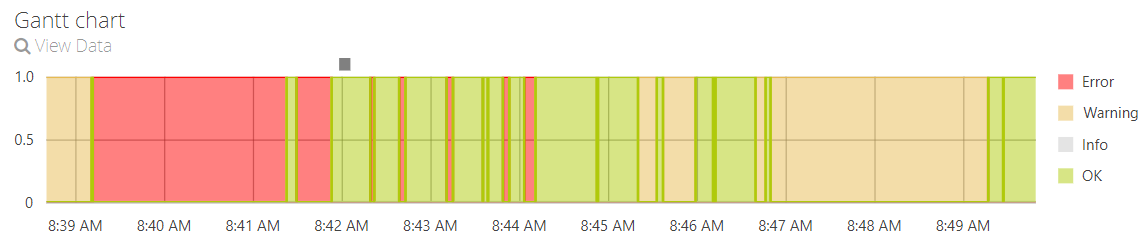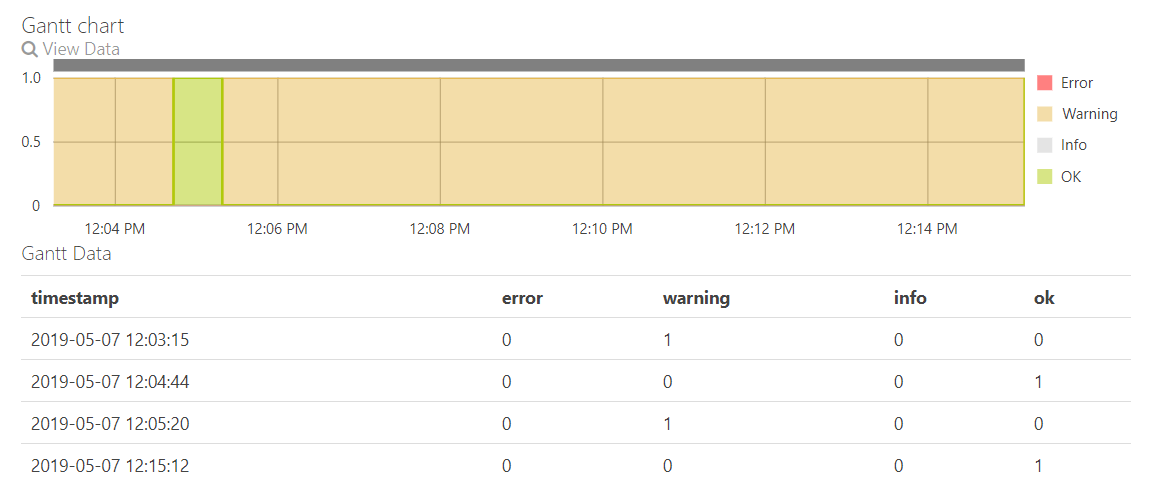Question:

How can I create a single row gantt chart e.g. to display the state of a machine?

Solution:

At the moment there is no "gantt chart" widget which you can choose, but with a little trick and some data preparation you can create a chart which looks like the one shown below:So how can you create this chart?

This is an "Area chart" with "Step Area" as line type and a series for each type of state. To make this working correct the input data has to be in a special format. To understand this format easily we will describe it for the simple example shown below:The input data has 5 columns. One for the timestamp of the state change and one for each state type. For each observation/row in the data only one of these state type columns is allowed to have value 1. All other columns have to have value 0. This is to avoid overlaps. Value 1 means that the state of the machine changed to this state. For example row 3 shows that at timestamp "2019-05-07 12:05:20" the state changed to "warning".

To get input data in this format you could either use an Analytics Query with calculated columns (if you have explicit state information) or you can also use the functions which are presented in the article "Set operations on time interval information (like alarms, warnings etc.)" (if you have implicit state information).

Using implicit state information:

If you have only implicit information on the machine state like alarm messages with start and stop times you can use the set operation functions in combination with the following code snippet.

Where the data frame 'df' contains the interval informations (category, start, stop) with three different categories where "e" stands for error, "w" stands for warning and "i" stands for information. In this code snipped we calculated the non-overlapping periods with the given priorization and assumed that if there is no error, warning or information the machine is ok.

#import the required packages
import datetime as dtime
from dateutil import tz

#do not forget to also import the function "nonoverlapping_periods" and "periods2events"
periods = nonoverlapping_periods(df, prio = ['e','w','i'])

event_list = periods2events(periods).drop('parallels', axis = 1) # df.loc[:,df.columns != 'parallels'] does the same as 'drop'

event_list['category'] = event_list['category'].astype('str')
event_list['error'] = ((event_list['category'] == 'e') & (event_list['edge'] == 1)).astype('int')
event_list['warning'] = ((event_list['category'] == 'w') & (event_list['edge'] == 1)).astype('int')
event_list['info'] = ((event_list['category'] == 'i') & (event_list['edge'] == 1)).astype('int')
event_list['ok'] = ((event_list['error'] == 0) & (event_list['warning'] == 0) & (event_list['info'] == 0)).astype('int')

timestamp = dtime.datetime.fromtimestamp(event_list['timestamp']/1000).astimezone(tz.gettz('Europe/Vienna')).strftime('%Y-%m-%d %H:%M:%S')
error = event_list['error']
warning = event_list['warning']
info = event_list['info']
ok = event_list['ok']